# 通常的做法

• 实现太麻烦。设计师给的设计稿通常是 px 为单位标注的，我们需要仔细的计算宽度和高度的比例，然后小心处理页面的布局。
• 难以处理屏幕宽高比与设计图不符时，带来的元素变形。所以最后展示的屏幕不能和设计稿的屏幕的宽高比差距太大。

# 理想的效果

• 屏幕尺寸和设计稿比例(16:9)一致时，占满屏幕

• 屏幕尺寸比设计图比例瘦时，上下留白，左右占满，并上下居中, 显示的比例保持16：9

• 屏幕尺寸比设计图比例胖时，左右留白，上下占满，并左右居中, 显示的比例保持16：9

# rem 方案

rem (font size of the root element), 是 css3 的引入的一个大小单位。即相对于根元素的 font-size 值的大小。所谓根元素在网页里一般就是 html. 举例说明：下例中，html 的 font-size 大小是 20px, 那么 1.4rem 和 2.4 rem 就分别代表着 28px 和 48px 了。

    html { font-size: 20px; }
test1 {
width: 1.4rem; //1.4 × 20px = 28px
}
test2 {
height: 2.4rem; //2.4 × 20px = 48px
}


  .doughnut {
top: 150px;
left: 30px;
width: 400px;
height: 300px;
}


  .doughnut {
top:  .781rem;     // 150 / 192 = 0.781
left: .156rem;     // 30  / 192 = 0.156
width: 2.083rem;   // 400 / 192 = 2.083
height: 1.563rem;  // 300 / 192 = 1.564
}



实际长度(px) = rootFontSize (html 的 font-size) * rem 长度



• 所有长度的比例必然和设计图一致。
• 实际的显示长度完全由 html 的 font-size 值决定（线性关系）。

1. 对于任意2条线，其实际长度的比例为 $\frac {x_1 f_s} z$ ： $\frac {x_2 f_s} z$ 就等于 他们在设计图上的比例 $x_1$ : $x_2$
2. 对于任意一条线，$x$ 和 $z$ 是固定值，其实际值随着 $f_s$ 值线性关系变化的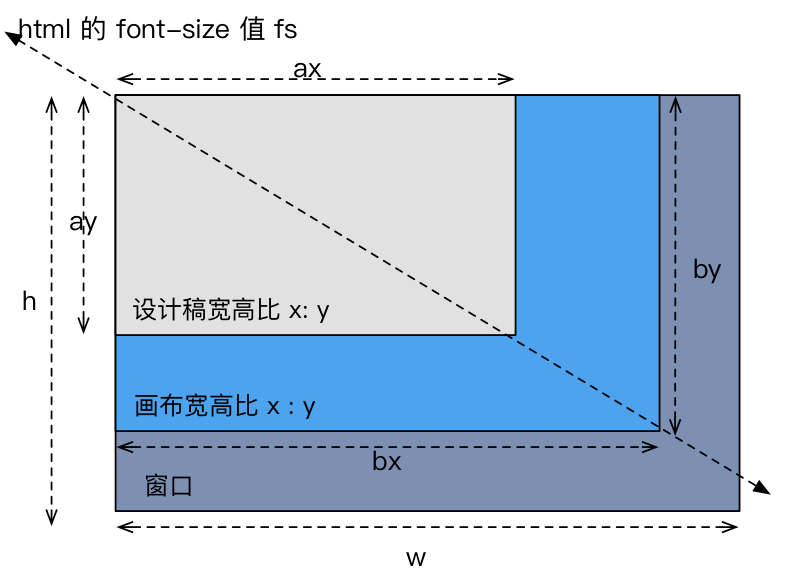• 设计稿的宽高分别为 $ax$ 和 $ay$, 比例为 $x$ : $y$.
• 实际显示的范围（我们称其为画布）比例和设计稿保持一致，所以可以设实际显示的画布的宽高为$bx : by$, 且所有线的实际显示长度是由 html 的 font-size 值 $f_s$ 线性决定的。
• 屏幕的尺寸不确定，假设其宽度为 $w$, 高度为 $h$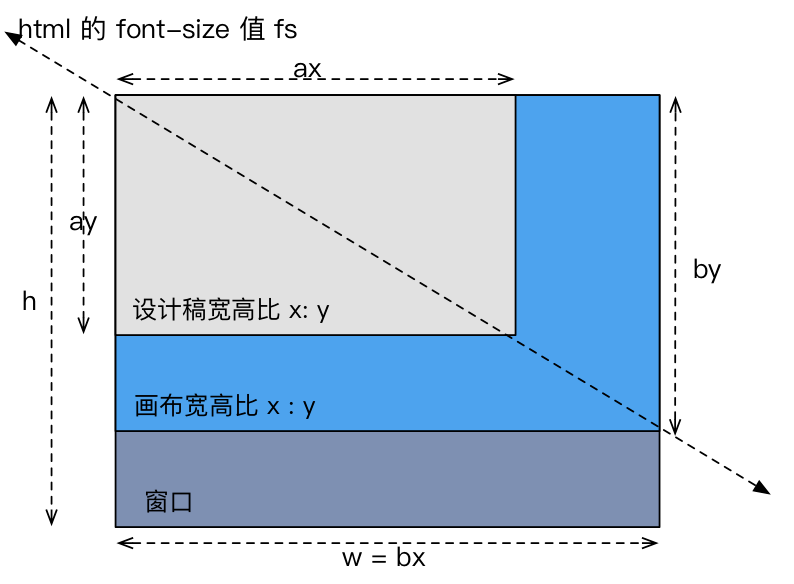# 我的方案

• 屏幕（窗口）尺寸和设计稿比例($x:y$)一致时，占满屏幕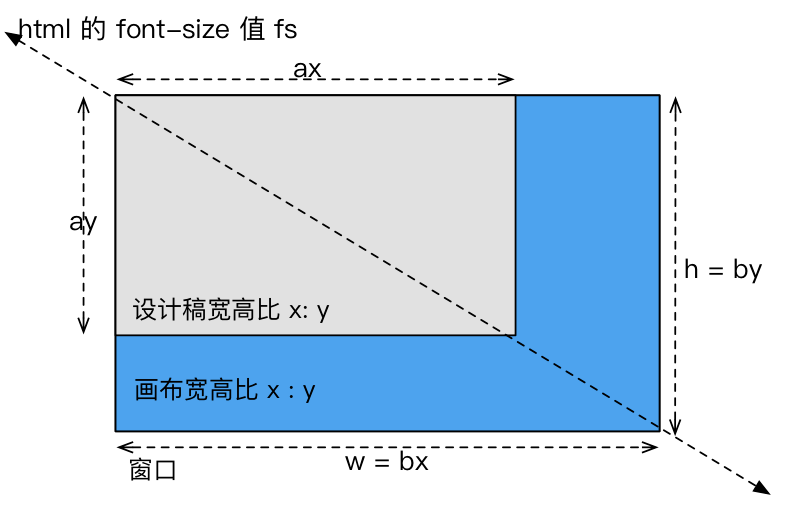这种情况肯定没问题，屏幕和真实页面完美重合.

• 屏幕（窗口）尺寸比设计图比例瘦时，上下留白，左右占满，并上下居中, 显示的比例保持($x:y$)这种情况也没问题，真实页面高度小于屏幕，然后页面内容上下居中就可以了。

• 屏幕（窗口）尺寸比设计图比例胖时，左右留白，上下占满，并左右居中, 显示的比例保持($x:y$)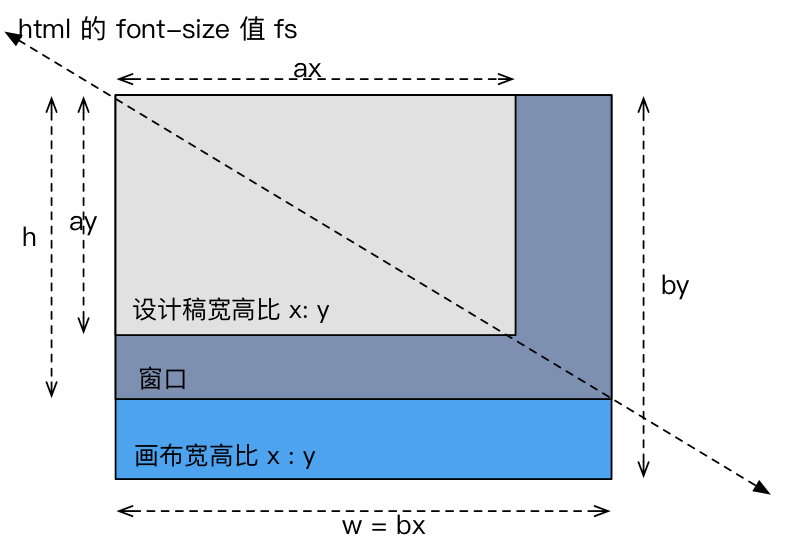问题出现了，在这种场景下，页面的高度超出了屏幕的高度，这就会导致垂直滚动条了。这就是我的方案处理的地方了。在这种场景下，我们需要页面的高度缩小为屏幕的高度，当然为了保持比例页面的宽度等也要等比例缩小.换句话说我们要把所有的线的长度等比例缩小，缩小后画布的高度要等于屏幕的高度，即下图所示的状态: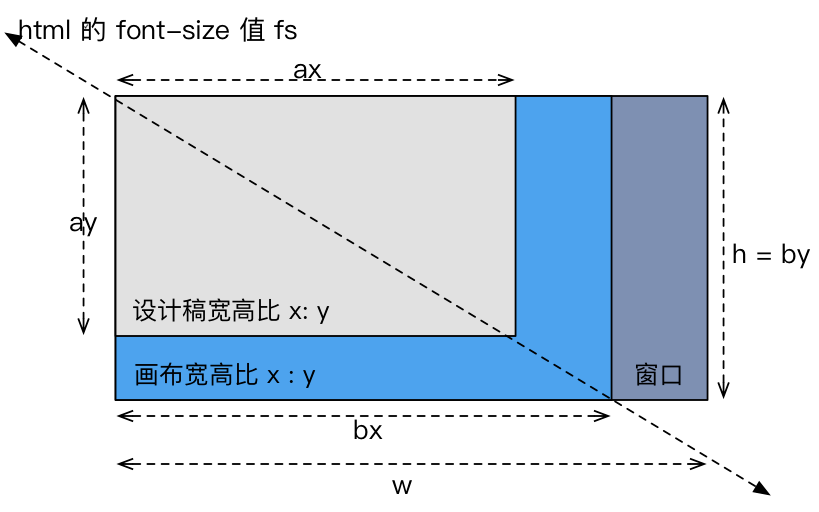${\frac {xh} {qy {\frac wq}}} = {\frac {xh} {yw}} = {\frac xy} / {\frac wh}$, 即 $\frac {设计稿宽高比} {窗口宽高比}$

Bingo. 综上可知: 当窗口尺寸比设计图比例胖时，只要我们在原来 $f_s$ 值的基础上，乘上 $\frac {设计稿宽高比} {窗口宽高比}$ 的缩小系数，就可以实现我们想要的效果了。

# 工程应用

1. 在 css 表示长度的时候，用设计稿上的长度除以 192, 算得 rem 的值。
2. 页面内写一段 js 代码，根据我们上面的公式去计算并设置 html 元素的 font-size 值。

# 示例

talk is cheap, show me the code

iframe 太小所以 echart 里的图会挤在一起看不清, 但是整个页面确实是能够保持对屏幕的适应的。

# 总结

https://github.com/njleonzhang/flexible-pc-full-screen https://github.com/QuellingBlade/postcss-px-to-rem https://github.com/QuellingBlade/lib-flexible-for-dashboard https://github.com/QuellingBlade/html-webpack-inline-plugin